• 目标：分析学生成绩的影响因素 1.导入原始数据，以及需要用到的库 import pandas as pd import numpy as np df = pd.read_csv('StudentsPerformance.csv') (数据来源于kaggle) 2.查看文件 从上面的信息可以看出这...
目标：分析学生成绩的影响因素
1.导入原始数据，以及需要用到的库
import pandas as pd
import numpy as np
df = pd.read_csv('StudentsPerformance.csv')

(数据来源于kaggle)
2.查看文件从上面的信息可以看出这一千个学生的数据中是没有空值，而且可以看出各列数据的类型。
还可以通过unique来查看某列数据都有哪些值，是否有无效数据。
df['parental level of education'].unique()
df['test preparation course'].unique()再来查看成绩是否为有效值（使用loc）
df.loc[(df['math score']<0) | (df['math score']>100) | (df['reading score']<0) | (df['reading score']>100) | (df['writing score']<0) | (df['writing score']>100)]可以看出成绩中没有无效值
3.数据处理
根据目标，删除一些不需要的列（使用drop）
df.drop(['gender','race/ethnicity'],axis=1)
#参数axis默认值为0，指行，要删除列的话将axis设为1
df_1 = df.drop(['gender','race/ethnicity'],axis=1)求学生成绩平均值
df_1['average']= round((df_1['math score']+df_1['reading score']+df_1['writing score'])/3)
#round()用来对数据四舍五入4.分析学生成绩影响因素
df_1.groupby('parental level of education')['average'].agg([np.mean]).plot.bar()
df_1.groupby('lunch')['average'].agg([np.mean]).plot.bar()
df_1.groupby('test preparation course')['average'].agg([np.mean]).plot.bar()从上面三张图可以初步得出结论：
1.父母学历越高，学生成绩越好
2.午餐吃的好的同学成绩较高
3.考试准备充分的同学成绩较高
以下，用相关系数分析父母学历对哪科成绩影响最大
先按照父母学历高低将表中数据替换为数值
df_1.replace({'some high school':1, 'high school':2,'some college':3,"associate's degree":4,"bachelor's degree":5,"master's degree":6},inplace = True)
#inplace = True是为了改变文档的源数据用corr求出相关系数（相关系数接近0，说明相关性小，越接近1，正相关性越强，越接近-1,负相关性越强）根据父母学历与学生数学、阅读、写作成绩的相关系数，可以看出，父母学历与三者都成正相关，其中与写作成绩相关性最强，而且各科之间相关性都很强，说明某一科成绩好的同学其他科成绩很可能也好。
5.结论
通过以上分析，可以初步得出以下的结论：
1.父母学历越高，学生成绩越好（对写作成绩的影响最大）
2.午餐吃的好的同学成绩较高
3.考试准备充分的同学成绩较高
4.某科成绩好的学生其他科成绩也较好（其中写作与阅读成绩相关性最强）


展开全文• 第四章 Python数据分析实战 习题（数据见附件sizhang.xlsx） 班主任现有一班级的两张表，如下。 表一：成绩表 学 号 C# 线 代 Python 16010203 78 88 96 表二：信息表 姓 名 学 号 手机号码 张三 16010203 ...
第四章 Python数据分析实战 习题（数据见附件sizhang.xlsx）

班主任现有一班级的两张表，如下。

表一：成绩表
学  号	C#	线  代	Python
16010203	78	88	96
表二：信息表
姓  名	学  号	手机号码
张三	16010203	16699995521
现请帮班主任做如下工作：

给成绩表加上姓名列；

给成绩表加上字段“总分”列，并求出总分；

增加列字段“等级”，标注每人“总分”的“优、良、中、及格、差”(270≤优，240≤良， 210≤中，及格≤180，差≤180)；

计算各门课程的平均成绩以及标准差；

做"总分"成绩分布图，纵坐标表示成绩，横坐标表示学号或者姓名，画出总分的均分横线，让每位同学的总分圆点分布在均分线上下，以便于观察每位同学的成绩离开均分的距离。

你们要的Python作业来啦，详细代码介绍可查看我徒弟的博客： Python数据分析实战
此处只是将我徒弟的代码做一个总合。其中有需要注意的地方，运行时有个问题: 读取Excel表格数据时，sheet_name根据你的表格sheet名称填写。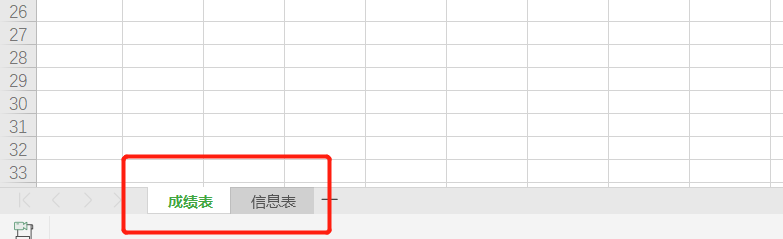在此声明，此代码来自我徒弟：潇囧囧_的博客，更多好文章，学习总结可关注我徒弟一起学习交流。
import pandas as pd
sheet1 = pd.read_excel("D:\Downloads\python_data.xlsx",sheet_name="成绩表")
sheet2 = pd.read_excel("D:\Downloads\python_data.xlsx",sheet_name="信息表")
print(sheet1)

sheet1 = pd.merge(sheet1,sheet2,left_on='学号',right_on='学号')      #连接两个表
sheet1 = sheet1.drop(columns = ['手机号'])
print(sheet1)

sheet1["总分"] = sheet1["C#"]+sheet1["线 代"]+sheet1["Python"]
print(sheet1)

def return_ans(x):

if x>=270:
b='优'
elif x>=240 and x<270:
b='良'
elif x>=210 and x<240:
b='中'
elif x>=180 and x<210:
b='及格'
else:
b='差'
return b
sheet1['等级'] = sheet1['总分'].apply(lambda x:return_ans(x))
print(sheet1)

print("C#的平均成绩为",sheet1['C#'].values.mean())
print("C#的标准差为",sheet1['C#'].values.std())
print("线 代的平均成绩为",sheet1['线 代'].values.mean())
print("线 代的标准差为",sheet1['线 代'].values.std())
print("Python的平均成绩为",sheet1['Python'].values.mean())
print("Python的标准差为",sheet1['Python'].values.std())

import matplotlib.pyplot as plt
plt.rcParams['font.sans-serif'] = ['Simhei']
s = sheet1['总分'].values.mean()
plt.bar(range(len(sheet1['总分'])),sheet1['总分'],width=0.8)
plt.xticks(range(len(sheet1['总分'])),sheet1['姓名'])
plt.axhline(s, color='red', linestyle='--')
plt.show()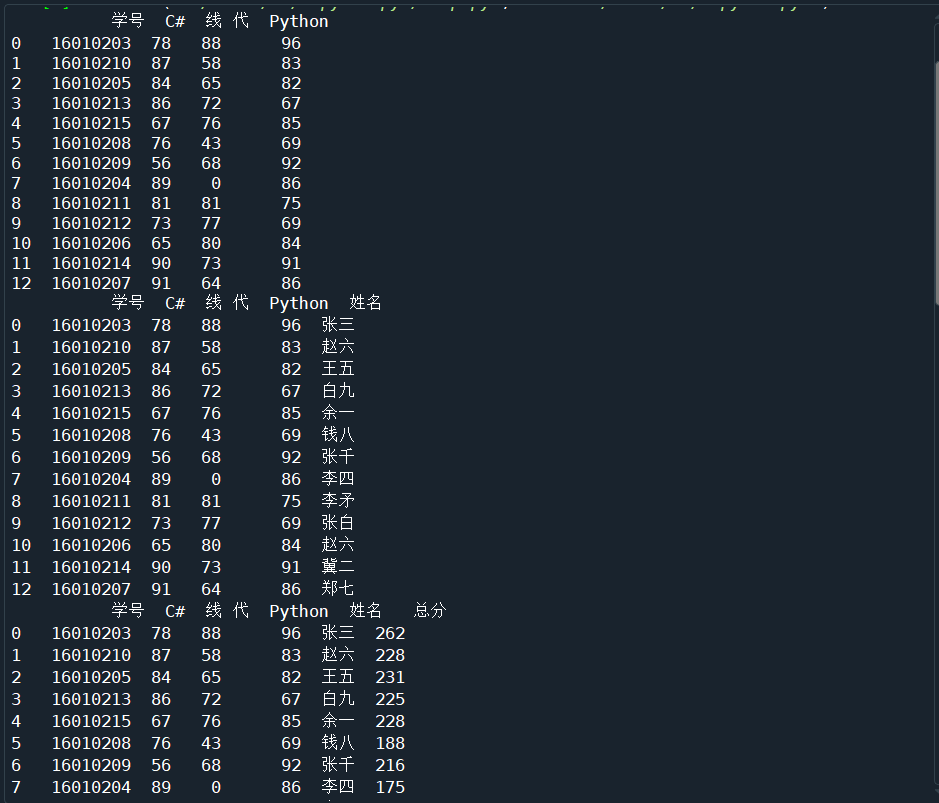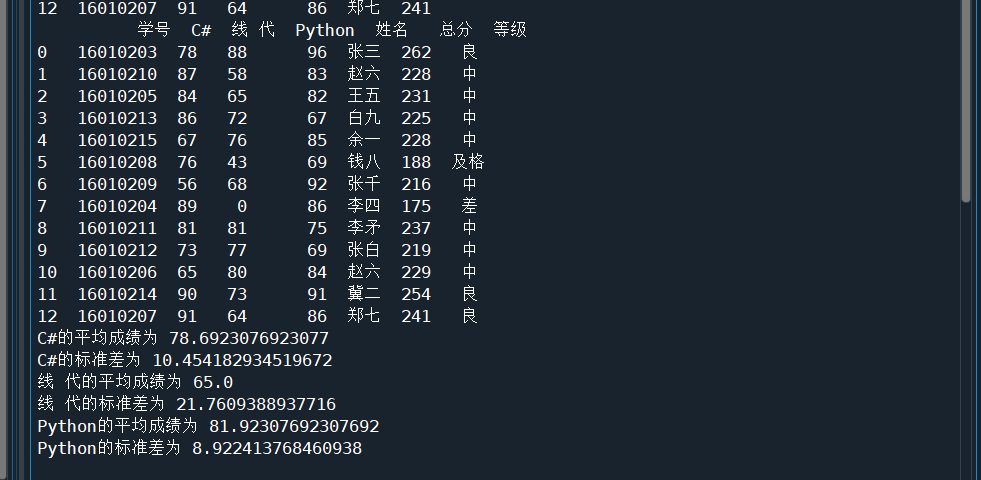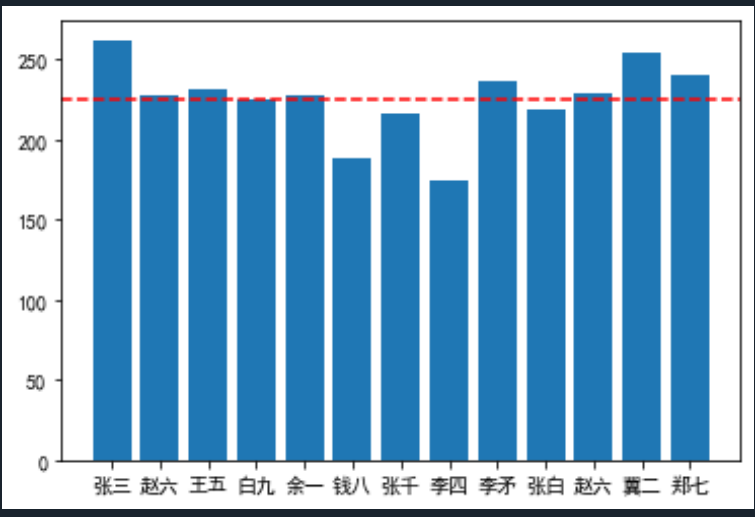欢迎关注公众号【夜来疏影】展开全文• python数据分析与机器学习课程 梯度下降与逻辑回归章节 成绩与录取 数据集excel版本
• 逛知乎的时候看到这样一个提问，Python自动化办公能做那些有趣或者有用的...为了更方面大家去学习，今天我将以学生考试成绩为例，手把手教你使用Python完成自动化办公，一键生成学生成绩数据分析报告(word版)，如果...

逛知乎的时候看到这样一个提问，Python自动化办公能做那些有趣或者有用的事情?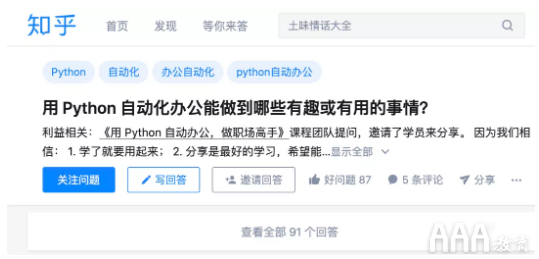看了一下这个提问，我想这可能是很多职场人面临的困惑，想把Python用到工作中来提升效率，却不知如何下手?Python在自动化办公领域越来越受欢迎，重复性工作让Python去做将是一种趋势。

看了一些办公自动化的文章，感觉更多是知识的罗列，看完后不知所云。为了更方面大家去学习，今天我将以学生考试成绩为例，手把手教你使用Python完成自动化办公，一键生成学生成绩数据分析报告(word版)，如果对PPT、excel自动化办公感兴趣，可以下方留言。

准备数据

没有数据，数据分析报告无从谈起。在5款优质的Python小工具，最后一款真神器!faker可以一行代码实现数据的生成，今天我将使用此方式。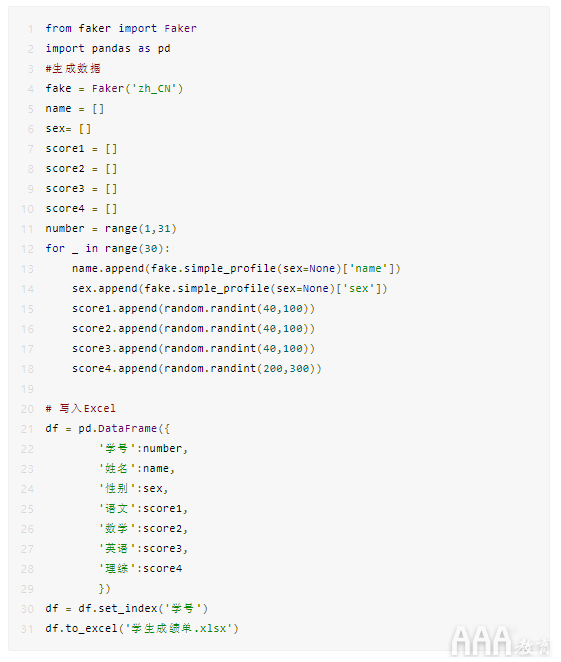生成分析报告

考试成绩明细

核心代码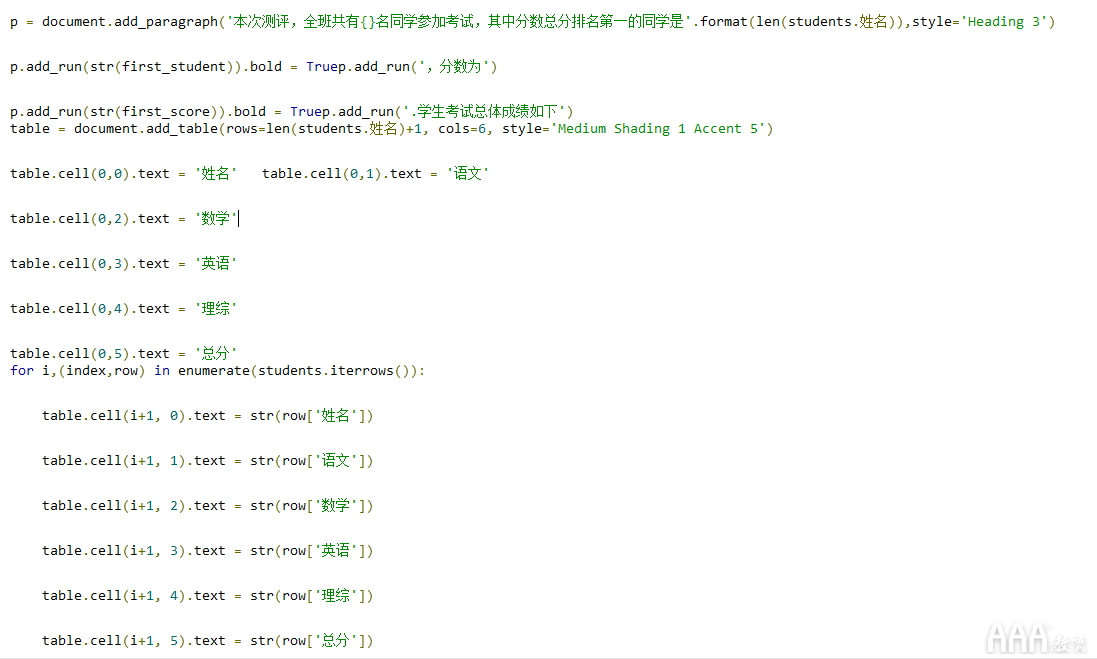结果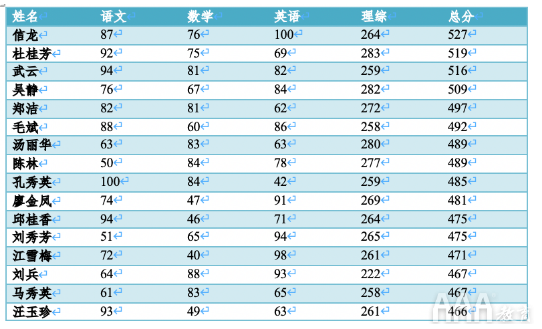考试成绩汇总

核心代码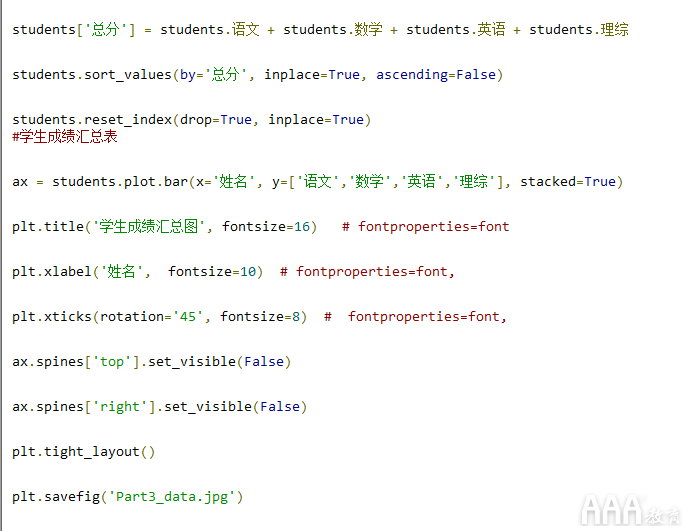结果展示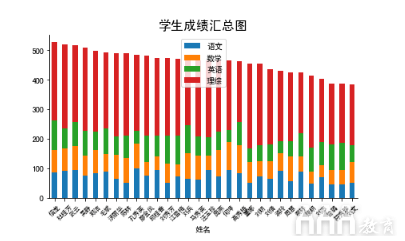各科成绩表现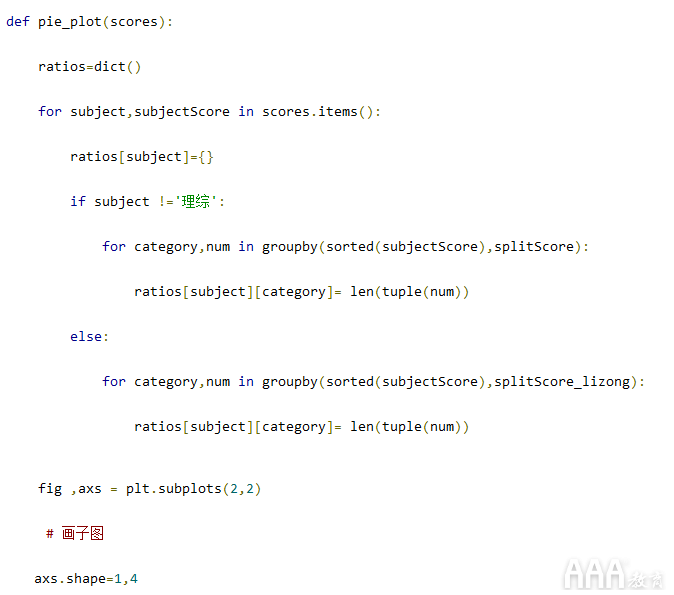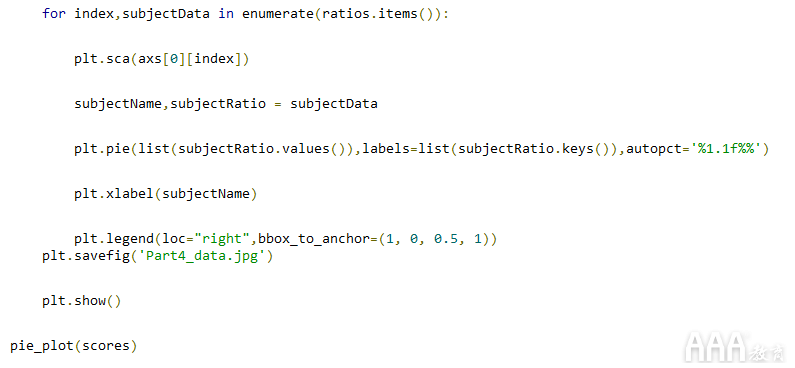结果展示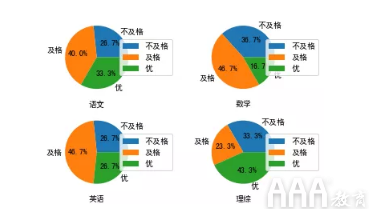历次考试成绩

上面都是对一个班级整体的数据分析报告。如果希望一份分析报告中，除了班级整体成绩分析外，还想生成一份针对个人成绩报告，目的不仅是可以知道这个班级的整体学习状况，也可以知道每个学生的学习情况，下面我们以一个王欢同学为例：

核心代码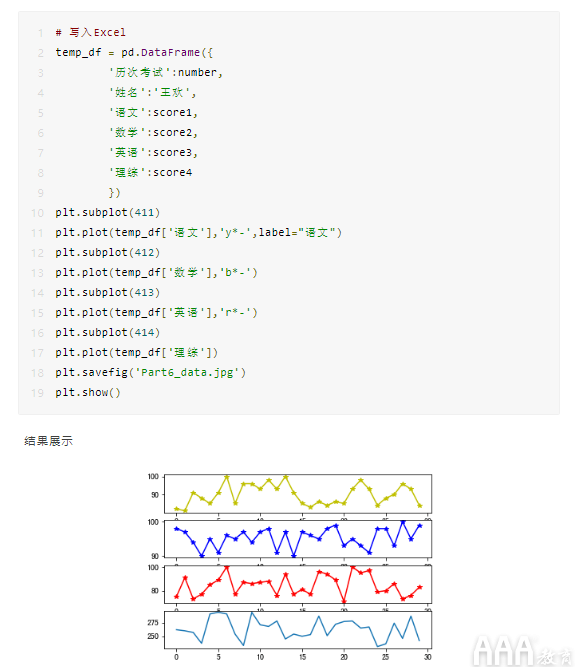该同学成绩概括性分析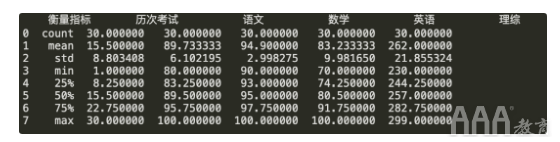成果展示

Python自动化办公，目的是提升效率，避免重复劳动，同时可以针对每个人的情况，做到千人千面，省时省力。效率可以做到一键生成，非常给力。

转载自：https://www.aaa-cg.com.cn/?xmta7

展开全文人工智能 大数据 编程语言
• 由于在绘制过程中缺少真实数据，我在这里采用np.random.normal(a,b,c)生成的随机数绘制直方图，a为平均值，b为标准差，c为生成数据的个数。利用np.arange(a,b,c)确定直方图x轴的范围及间距，a为最小值，b为最大值，c...
1.直方图的绘制也需要用到matplotlib下的pylab，只不过在绘制折线图时我们采用的是plot()，而绘制直方图时我们需要采用hist()。由于在绘制过程中缺少真实数据，我在这里采用np.random.normal(a,b,c)生成的随机数绘制直方图，a为平均值，b为标准差，c为生成数据的个数。利用np.arange(a,b,c)确定直方图x轴的范围及间距，a为最小值，b为最大值，c为间距。用plt.hist(a,b)绘制，a为数据，b为直方图的特性，可有可无。import matplotlib.pylab as pltimport numpy as npda = np.random.normal(5.0, 0.5, 3000)dis = np.arange(3.5, 5, 0.1)plt.hist(da, dis)plt.show()2.绘制子图时，我们需要先将空间分为若干份，这时需要采用命令plt.subplot(a,b,c)，其中a表示行，b表示列，c表示从第一行开始从左向右数到c的当前区域。例如，如果想要在第一行绘制三个子图，第二行绘制一个子图，需要采用以下代码import matplotlib.pylab as pltimport numpy as npplt.subplot(2, 3, 1)plt.subplot(2, 3, 2)plt.subplot(2, 3, 3)plt.subplot(2, 1, 2)plt.show()3.区域拆分结束后我们该怎样在每个区域内绘制相应的图像呢？我们在前面用代码将区域拆分为了四部分，如果我们想在某个区域内绘图，只需将绘图代码写在那一部分的代码下即可import matplotlib.pylab as pltimport numpy as npplt.subplot(2, 3, 1)    #下面的语句绘制第一个子图x1 = [1, 3, 5, 7, 9, 11]y1 = [2, 4, 6, 8, 10, 12]plt.plot(x1, y1, 'c')plt.subplot(2, 3, 2)    #下面的语句绘制第二个子图x2 = [3, 5, 6, 7, 9, 13, 20]y2 = [1, 6, 2, 3, 5, 7, 11]plt.plot(x2, y2, 'ob')plt.subplot(2, 3, 3)    #下面的语句绘制第三个子图x3 = [2, 5, 7, 8, 10, 11]y3 = [3, 5, 4, 1, 15, 10]plt.plot(x3, y3, '-.')plt.plot(x3, y3, 's')plt.subplot(2, 1, 2)    #下面的语句绘制第四个子图da = np.random.normal(5.0, 0.5, 3000)dis = np.arange(3.5, 5, 0.1)plt.hist(da, dis)plt.show()展开全文• 案例:该数据集的是一个关于每个学生成绩数据集,接下来我们对该数据集进行分析，判断学生是否适合继续深造 数据集特征展示 1 GRE 成绩 (290 to 340) 2 TOEFL 成绩(92 to 120) 3 学校等级 (1 to 5) 4 自身的意愿 (1...
• 利用Python分析学生成绩一、题目描述二、解题步骤运行环境题目分析三、完整代码四、运行结果五、参考文献 一、题目描述 个人构造一个30名学生的班级，每名学生有三门课程，学生的学号和各门成绩形如： 学号 高数 ...csv
• ## Python数据分析实战

千次阅读 多人点赞 2020-06-11 14:42:48
Python数据分析实战 数据及要求 现请帮班主任做如下工作： 给成绩表加上姓名列； 给成绩表加上字段“总分”列，并求出总分； 增加列字段“等级”，标注每人“总分”的“优、良、中、及格、差”(270≤优，240...可视化
• 问题描述 代码实现 参考文章 8行Python代码实现excel两个sheet表合并 用Python将同一个excel中的多个sheet合并成一个sheet python读取excel指定列名，dataframe连接，两列相减作为第三列的值
• Pandas是什么？ 1、一个强大的分析结构化数据的工具集 2、基础是NumPy，提供了高性能矩阵的运算 ...比如，学生成绩分析，股票数据分析等 4、提供数据清洗功能 Pands数据结构，主要分为两种，Series和DataFrame
• Python语言可以利用Pandas、Pyecharts等各种类库，进行数据分析。 本文介绍的成绩分析大体分为三步： 一、拼合单科成绩，合成学年成绩，计算总分，按总分成绩排名次，然后由学年成绩筛选出各个班级的成绩，将学年......

# python成绩数据分析python 订阅